Electron. J. Diff. Equ., Vol. 2015 (2015), No. 283, pp. 1-11.

### Exponent of convergence of solutions to linear differential equations in the unit disc Nacera Berrighi, Saada Hamouda

Abstract:
In this article, we study the exponent of convergence of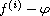where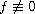is a solution of linear differential equations with analytic and meromorphic coefficients in the unit disc and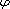is a small function of. From this results we deduce the fixed points of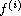by taking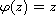. We will see the similarities and differences between the complex plane and the unit disc.

Submitted May 21, 2015. Published November 11, 2015.
Math Subject Classifications: 34M10, 30D35.
Key Words: Linear differential equations; exponent of convergence; growth of solutions, unit disc.

Show me the PDF file (247 KB), TEX file, and other files for this article.Nacera Berrighi Laboratory of Pure and Applied Mathematics University of Mostaganem, UMAB, Algeria email: berrighinacera@univ-mosta.dz Saada Hamouda Laboratory of Pure and Applied Mathematics University of Mostaganem, UMAB, Algeria email: hamoudasaada@univ-mosta.dz, hamouda_saada@yahoo.fr